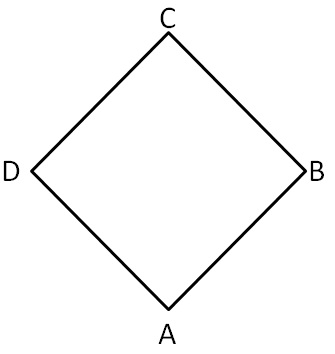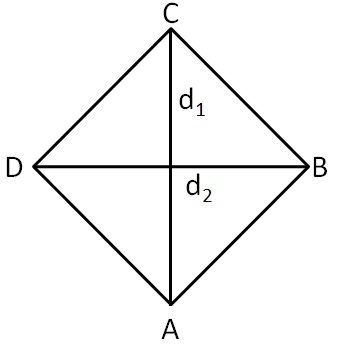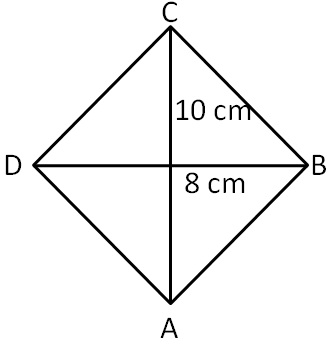Area of Rhombus

Chapter 9 Class 8 Mensuration
Concept wise

A rhombus is a parallelogram with all sides equal

To find area of rhombus,

We draw its diagonalsSo,

Area of Rhombus ABCD

= 1/2 × d 1 × d 2#### Find area of rhombus ABCDGiven Diagonals,

d 1 = 10 cm

& d 2 = 8 cm

Now,

Area of rhombus = 1/2 × d 1 × d 2

= 1/2 × 10 × 8

= 10 × 4

= 40 cm 2

Learn in your speed, with individual attention - Teachoo Maths 1-on-1 Class